### Introduction

Support Vector Machine (abbreviated as SVM) is a supervised machine learning algorithm that can be used for both classification and regression problems (mainly classification). SVM’s approach to classification is to create a plane of best fit that can accurately distinguish between the class labels in a given dataset. In other words, for any n-dimensional data, SVM creates an n-1 dimensional hyperplane (a hyperplane is a higher dimensional generalization of lines and planes) which acts as a decision boundary for any given dataset.

##### Categories of SVM

The types of Support Vector Machines are based on the separability of data. These types have been explained with graphs with the height of boys and girls on the x-axis and their hair length on the y-axis:

1. Linear SVM: If the data is linearly separable, the linear SVM approach can be efficiently applied.2. Non-linear SVM: If there is no linearly separable hyperplane possible, the non-linear approach can be taken wherein the points can be mapped to higher dimensionality using kernel functions to form a separable hyperplane.### Hyperplane Derivation

There are various algorithms that try to find a hyperplane for separating data (such as perceptron). However, SVM finds out the most optimal hyperplane that can accurately separate the various labels. The formula for hyperplane is as follows: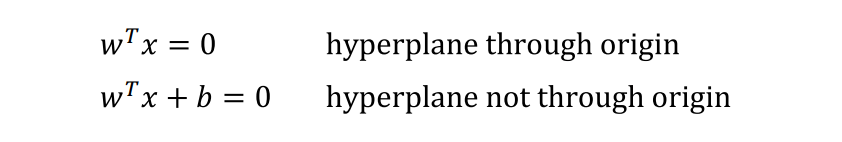Here,

• w is a set of coefficients. It can be considered as a vector perpendicular to the hyperplane.
• b is the bias value. If b > 0, the hyperplane is towards w, and if b < 0, the hyperplane is in the opposite direction of w.

In order to make the derivation of the hyperplane, here are some of the assumptions taken:

• The dataset has two-dimensional data.
• The dataset has linearly separable values.

The figure below depicts how a two-dimensional linearly separable data looks like. The red dots indicate negative values and the green crosses indicate positive values.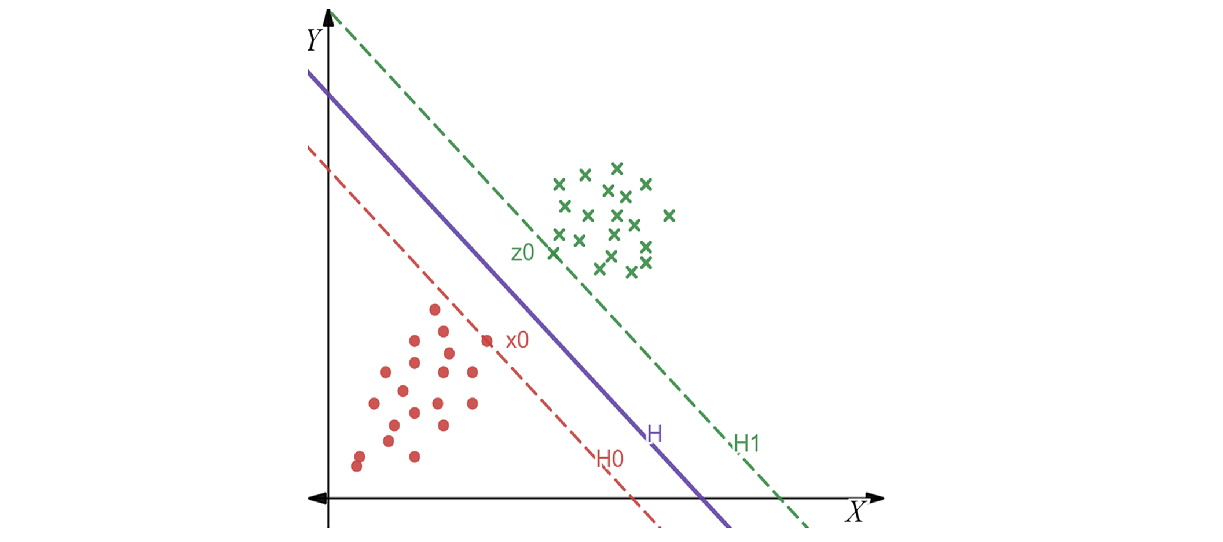We must choose a hyperplane that satisfies the following conditions:

• The margin must be maximum.
• The classification of labels must be accurate.

Each point in the diagram above can be considered to be a vector from the origin. Let us consider the hyperplane equation with bias value to be the equation for our optimal hyperplane. The optimal hyperplane in the figure above is H, the hyperplane towards the negative side is H0 and the hyperplane towards the positive side is H1. The equations are as follows: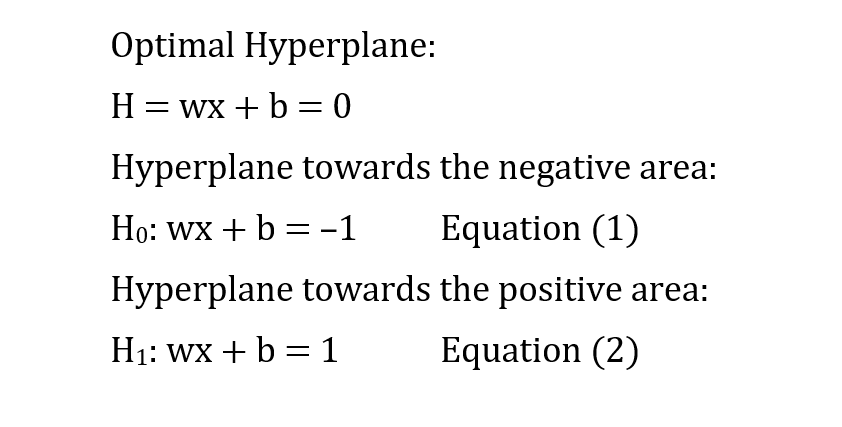Let x0 and z0 be a negative and a positive data point respectively which falls under the lines H0 and H1. These two points are the closest to our optimal hyperplane H. Let the distance between x0 and z0 is d. From the definition of w above, a perpendicular line from H has been drawn to depict w in the figure below: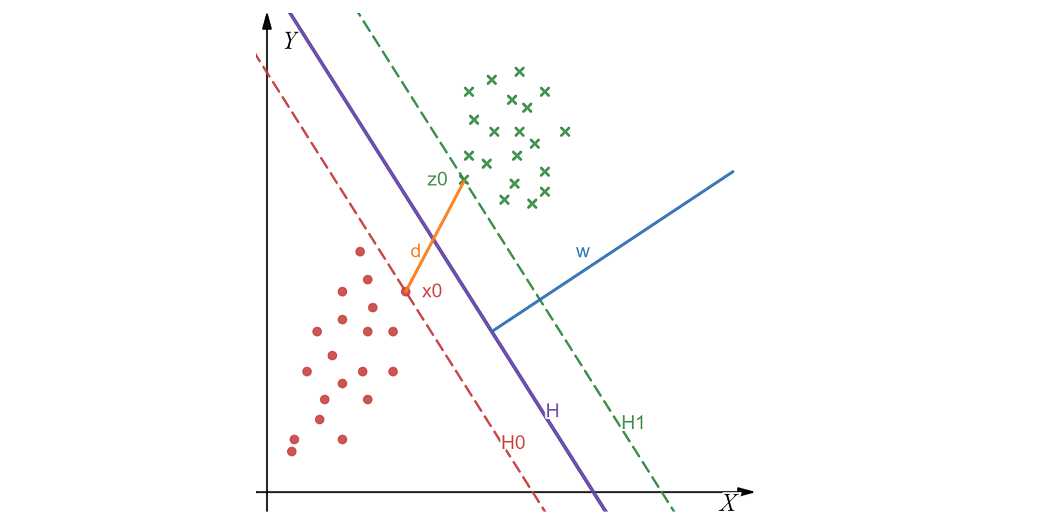From the figure above, it is clear that the support vectors are x0 and z0 whereas the distance d is the margin. Let us try to find the expression of margin.

#### Margin Expression

The distance d is a scalar quantity as it is just the distance between two points. However, x0 is a vector. In order to make d a vector, we must multiply it with a unit vector. Since w is perpendicular to H, H0, and H1, the w vector can be used to make d a vector as follows: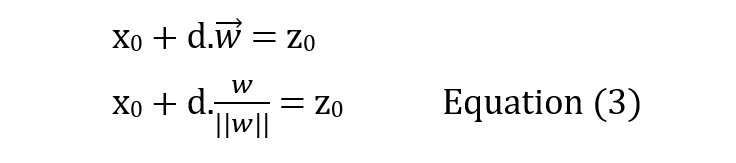Since z0 is a point in H1, it will satisfy the equation of H1 (equation (1)) as follows:This is the expression for margin in SVM. According to the conditions mentioned above, the margin (d) must be maximum. For d to have the maximum value, the hyperplane must have the smallest value of ||w|| in its equation. This leads us to formulate our objective function.

#### Objective Function

For the given set of assumptions, the objective function of the classifier will be to minimize ||w|| subject to the condition that all positive samples are above H1 and all negative samples are below H0. Mathematically it can be interpreted as: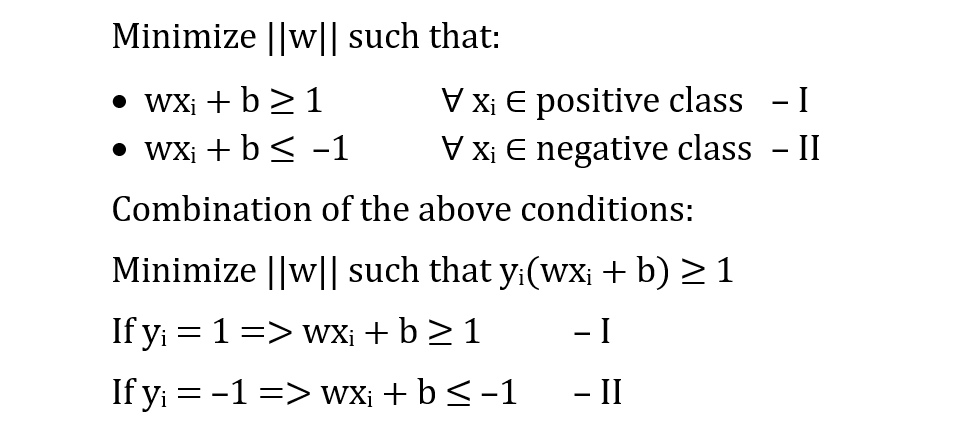It must be noted that ||w|| is not a convex function (which means that only the local maximum can be found for this function). This function is also undifferentiable. Therefore we find the equivalent quadratic function of ||w|| since quadratic functions are convex as well as differentiable. This will lead us to our constrained objective function.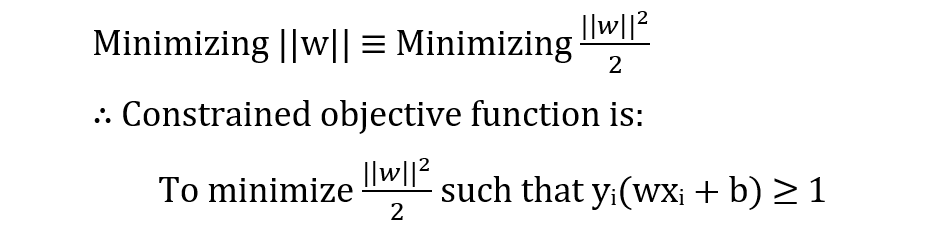Using the set of Lagrange multipliers, the constrained objective function can be written as: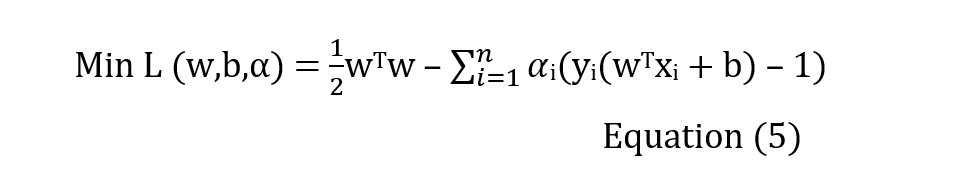In this equation, we minimize L with respect to w and b, and maximize with respect to alpha. The equation is currently in its primal form, which is very difficult and complicated to solve. This is because the primal form of the objective function in SVM has three variables. Therefore, we try to transform the function into its dual form. If the value of w and b needs to be minimum, then their respective partial differentiation with the function must be 0.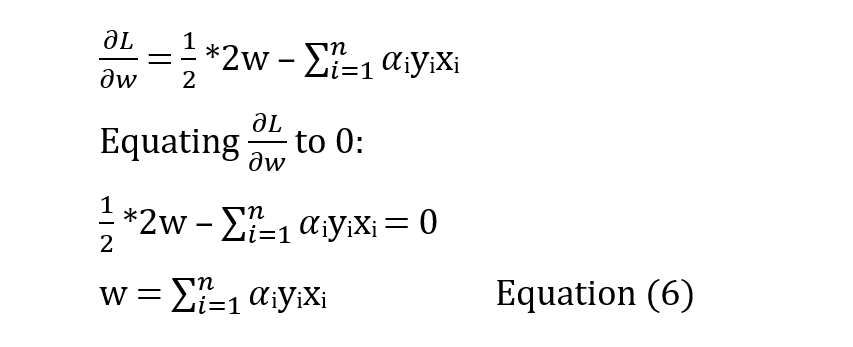From this equation, it can be inferred that the optimal value of w is a linear combination of the inputs and the outputs, scaled by the value of alpha.From this equation, it can be inferred that all the Lagrange multipliers of the positive class are equal to all the Lagrange multipliers of the negative class.

Using the Karush Kuhn Tucker (KKT) conditions in the theory of Lagrange multiplier optimizations, we get the following equation: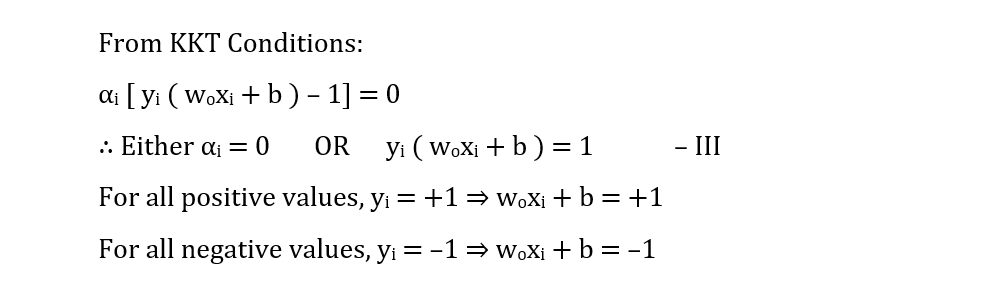However, the value of alpha will not be 0 only for the support vectors. Also, for the value of the hyperplane equation to be a singleton (either positive or negative) is only for the support vectors. Therefore, if the value of alpha is 0, that means the vector is not a support vector.The dual form requires only the value of alpha to generate the hyperplane derivation. Using the value of alpha, the solution for w and b can be found respectively using the KKT conditions. Once the value of w and b is known, the optimum hyperplane is generated.

### Pros and Cons of SVM

##### Pros
• The margin of separation is clear and the interface works accurately.
• Effective in high-dimensional spaces.
• The method is most effective when the number of dimensions is greater than the number of samples (and if dimensionality reduction has not been opted for the dataset).
• In the decision function, it uses a subset of training points (called support vectors), making it memory-efficient as well.
##### Cons
• Large data sets require a longer training time, so it does not perform well. In addition, it performs poorly when a lot of noise is present in the dataset.
• Probability estimates are not directly provided by SVM. Instead, they are calculated through expensive k-fold cross-validation.
• SVM requires scaling of data for varied ranges of values in a dataset.

### Implementation

We can use the weka library for S2 IDE to build a support vector classifier model. For this implementation, let us use the iris dataset that is present in ARFF format for weka to read and process it.

First, we import the dependencies and the classes present in the weka library. The support vector classifier method is present in the SMO class under the functions subpackage.

				
@file:Repository("https://mvnrepository.com/artifact/nz.ac.waikato.cms.weka/weka-stable")
@file:DependsOn("nz.ac.waikato.cms.weka:weka-stable:3.8.0")


				
import weka.core.Instances
import weka.classifiers.Classifier
import weka.classifiers.Evaluation
import weka.classifiers.functions.SMO
import weka.core.converters.ConverterUtils



We read the training dataset and convert it to a readable format for the library methods. Here is how the label of interest for this dataset has been indexed:

				
val sourcetrain = ConverterUtils.DataSource("train_data.arff")
val traindataset = sourcetrain.dataSet
traindataset.setClassIndex(traindataset.numAttributes() - 1)
val numClasses = traindataset.numClasses()
for (i in 0 until numClasses)
{
val classvalue = traindataset.classAttribute().value(i)
println("Class Value " + i + " is " + classvalue)
}



Output:

				
Class Value 0 is Iris-setosa
Class Value 1 is Iris-versicolor
Class Value 2 is Iris-virginica



We repeat the same thing work the testing dataset.

				
val sourcetest = ConverterUtils.DataSource("test_data.arff")
val testdataset = sourcetest.dataSet
testdataset.setClassIndex(testdataset.numAttributes() - 1)



We create an object of the classifier and build a model. We can also see the capabilities of the model.

				
val svc = SMO()
svc.buildClassifier(traindataset)
println(svc.getCapabilities().toString())



Output:

				
Capabilities: [Nominal attributes, Binary attributes, Unary attributes, Empty nominal attributes, Numeric attributes, Missing values, Nominal class, Binary class, Missing class values]
Dependencies: [Nominal attributes, Binary attributes, Unary attributes, Empty nominal attributes, Numeric attributes, Date attributes, String attributes, Relational attributes]
min # Instance: 1



We can find the predicted value for each test dataset value and compare it with the actual value.

				
println(String.format("Actual\t\tPredicted"))
for (i in 0 until testdataset.numInstances())
{
val actualclass = testdataset.instance(i).classValue()
val actual = testdataset.classAttribute().value(actualclass.toInt())
val newinst = testdataset.instance(i)
val pred = svc.classifyInstance(newinst)
val predstring = testdataset.classAttribute().value(pred.toInt())
println(String.format("$actual\t$predstring"))
}



Output:

				
Actual		Predicted
Iris-setosa	Iris-setosa
Iris-setosa	Iris-setosa
Iris-setosa	Iris-setosa
Iris-setosa	Iris-setosa
Iris-setosa	Iris-setosa
Iris-setosa	Iris-setosa
Iris-setosa	Iris-setosa
Iris-setosa	Iris-setosa
Iris-setosa	Iris-setosa
Iris-setosa	Iris-setosa
Iris-setosa	Iris-setosa
Iris-setosa	Iris-setosa
Iris-setosa	Iris-setosa
Iris-setosa	Iris-setosa
Iris-setosa	Iris-setosa
Iris-setosa	Iris-setosa
Iris-setosa	Iris-setosa
Iris-setosa	Iris-setosa
Iris-setosa	Iris-setosa
Iris-setosa	Iris-setosa
Iris-setosa	Iris-setosa
Iris-versicolor	Iris-versicolor
Iris-versicolor	Iris-versicolor
Iris-versicolor	Iris-versicolor
Iris-versicolor	Iris-versicolor
Iris-versicolor	Iris-versicolor
Iris-versicolor	Iris-versicolor
Iris-versicolor	Iris-versicolor
Iris-versicolor	Iris-versicolor
Iris-versicolor	Iris-versicolor
Iris-versicolor	Iris-versicolor
Iris-versicolor	Iris-versicolor
Iris-versicolor	Iris-versicolor
Iris-versicolor	Iris-versicolor
Iris-versicolor	Iris-versicolor
Iris-versicolor	Iris-versicolor
Iris-versicolor	Iris-versicolor
Iris-virginica	Iris-virginica
Iris-virginica	Iris-virginica
Iris-virginica	Iris-virginica
Iris-virginica	Iris-virginica
Iris-virginica	Iris-virginica
Iris-virginica	Iris-virginica
Iris-virginica	Iris-versicolor
Iris-virginica	Iris-virginica
Iris-virginica	Iris-virginica
Iris-virginica	Iris-virginica
Iris-virginica	Iris-virginica
Iris-virginica	Iris-virginica
Iris-virginica	Iris-virginica
Iris-virginica	Iris-virginica
Iris-virginica	Iris-virginica
Iris-virginica	Iris-virginica
Iris-virginica	Iris-virginica
Iris-virginica	Iris-virginica
Iris-virginica	Iris-virginica
Iris-virginica	Iris-versicolor
Iris-virginica	Iris-virginica
Iris-virginica	Iris-virginica



The following are the evaluation metrics for the given model that is built:

				
val evalsvc = Evaluation(traindataset)
evalsvc.evaluateModel(svc, testdataset)
System.out.printf("Correct: %.2f\n",evalsvc.pctCorrect())
System.out.printf("Incorrect: %.2f\n",evalsvc.pctIncorrect())
println(evalsvc.toMatrixString())
System.out.printf("Precision: %.2f\n", evalsvc.precision(1)*100)
System.out.printf("Recall: %.2f\n", evalsvc.recall(1)*100)
System.out.printf("F1 score: %.2f\n", evalsvc.fMeasure(1)*100)



Output:

				
Correct: 96.61
Incorrect: 3.39
=== Confusion Matrix ===

a  b  c   --- classified as
21  0  0 |  a = Iris-setosa
0 16  0 |  b = Iris-versicolor
0  2 20 |  c = Iris-virginica

Precision: 88.89
Recall: 100.00
F1 score: 94.12